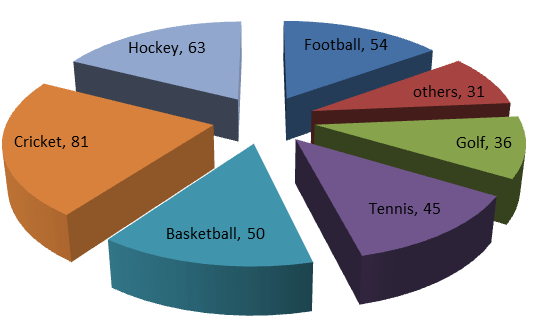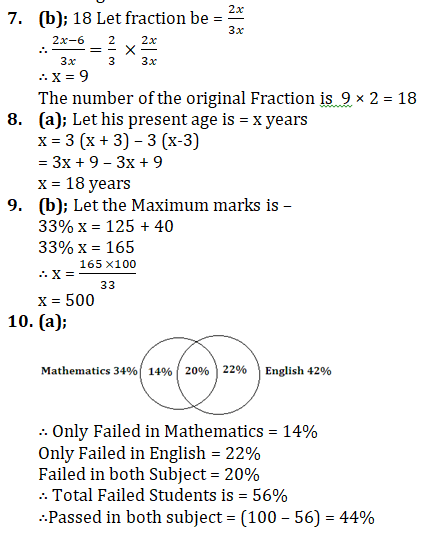# Quant Quiz for IBPS CLERK PRELIMS | 10th November 2018

Quant Quiz to improve your Quantitative Aptitude & Data Interpretation. For upcoming exams like Bank, SSC, Railway, UPSC, UPSSSC, CDS, UPTET, KVS, DSSSB and other Government exams.

Directions (1 to 5): Study the following information to answer the questions given below:

The given data shows in degrees.The pie-chart given above shows the expenditure of a country on various sports during a particular year.

Q1.  What percent of the total expenditure is spent on Tennis?

(a) 12  %

(b) 22  %

(c) 25%

(d) 45%

(e) None of these

Q2.  What percent more is spent on Hockey than on Golf?

(a) 27%

(b) 35%

(c) 37.5%

(d) 75%

(e) None of these

Q3. What percent less is spent on Football than on Cricket?

(a) 22 (2/9)%

(b) 27%

(c) 33 (1/3)%

(d) 37(1/2)%

(e) None of these

Q4. If the total amount spent on sports during the year was Rs.2 Crore, the amount spent on Cricket and Hockey together was?

(a) Rs.800000

(b) Rs.8000000

(c) Rs.12000000

(d) Rs.16000000

(e) None of these

Q5.  If the total amount spent on sports during the year be Rs.18000000, the amount spent on Basketball exceeds that on Tennis by

(a) Rs.250000

(b) Rs.360000

(c) Rs.375000

(d) Rs.410000

(e) None of these

Q6. The average weight of A, B and C is 45 Kg. If the average weight of A and B be 40 kg and that of B and C be 43 kg, then the weight of B is?

(a) 17 Kg

(b) 20   kg

(c) 26 Kg

(d) 31 kg

(e) None of these

Q7. The numerator and the denominator of a fraction are in the ratio of 2 : 3. If 6 is subtracted from the numerator, the result is a fraction that has value 2/3 of the original fraction. The numerator of the original fraction is

(a) 6

(b) 18

(c) 27

(d) 36

(e) None of these

Q8. A person was asked to state his age in years. His reply was, “Take my age three years hence, multiply it by 3 and then subtract three times my age three years ago.” What was the age of the person?

(a) 18 years

(b) 20 years

(c) 24 years

(d) 32 years

(e) None of these

Q9. A student has to obtain 33% of the total marks to pass. He got 125 marks and was declared failed by 40 marks. What were the maximum marks?

(a) 300

(b) 500

(c) 800

(d) 1000

(e) None of these

Q10. In an examination, 34% of the students failed in Mathematics and 42% failed in English. If 20% of the students failed in both the subjects, the percentage of students who passed in both the subjects was

(a) 44%

(b) 50%

(c) 54%

(d) 56%

(e) None of these

## Solutions